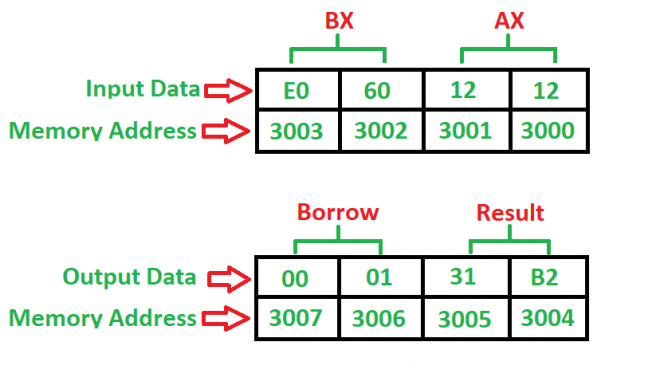# 8086 program to subtract two 16-bit numbers with or without borrow

• Last Updated : 22 May, 2018

Problem – Write a program to subtract two 16-bit numbers where starting address is 2000 and the numbers are at 3000 and 3002 memory address and store result into 3004 and 3006 memory address.

Example –Algorithm –

1. Load 0000H into CX register (for borrow)
2. Load the data into AX(accumulator) from memory 3000
3. Load the data into BX register from memory 3002
4. Subtract BX with Accumulator AX
5. Jump if no borrow
6. Increment CX by 1
7. Move data from AX(accumulator) to memory 3004
8. Move data from CX register to memory 3006
9. Stop

Program –

Memory Mnemonics Operands Comment
2000 MOV CX, 0000 [CX] <- 0000
2003 MOV AX,  [AX] <- 
2007 MOV BX,  [BX] <- 
200B SUB AX, BX [AX] <- [AX] – [BX]
200D JNC 2010 Jump if no borrow
200F INC CX [CX] <- [CX] + 1
2010 MOV , AX  <- [AX]
2014 MOV , CX  <- [CX]
2018 HLT Stop

Explanation –

1. MOV is used to load and store data.
2. SUB is used to subtract two numbers where their one number is in accumulator or not.
3. JNC is a 2-bit command which is used to check whether the borrow is generated from accumulator or not.
4. INC is used to increment an register by 1.
5. HLT is used to stop the program.
6. AX is an accumulator which is used to load and store the data.
7. BX, CX are general purpose registers where BX is used for storing second number and CX is used to store borrow.
My Personal Notes arrow_drop_up
Recommended Articles
Page :